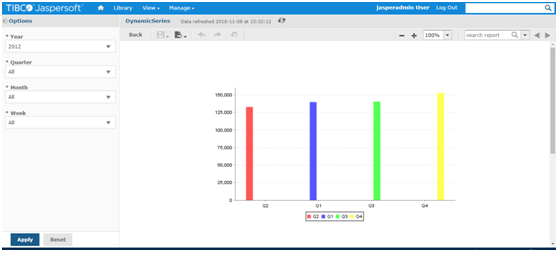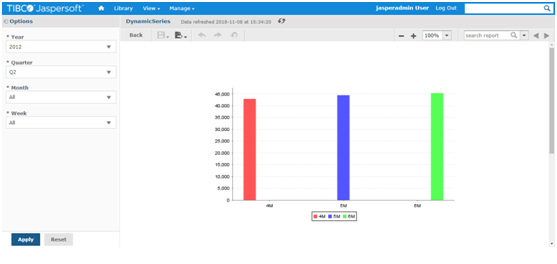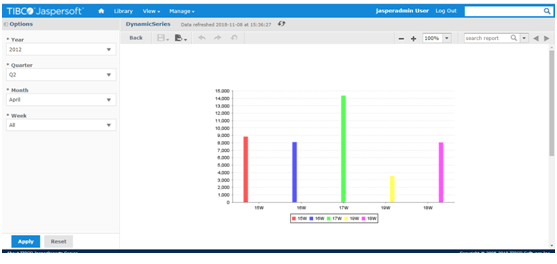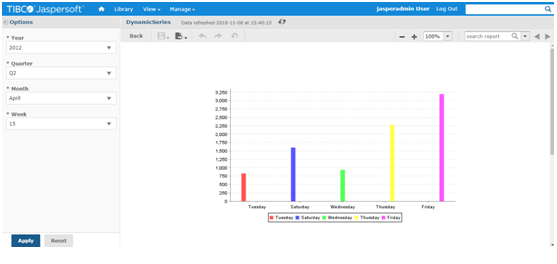# Dynamically Series Changing for Bar Chart based on Parameter Selection In Jasper

#### Posted on November 9, 2018 by By admin, in Jaspersoft | 0

Prerequisites: Jasper Server 7.1, Jasper Studio 6.3
Database: Foodmart

We have come across one requirement like whenever user select

Year the Bar chart should show Quarter Wise sales data
Quarter the Bar Chart should show Month Wise sales data
Month the Bar Chart should show Week wise sales data
Week the Bar Chart should show Day wise sales data

We have achieved this functionality by SQL query

```select case when
(\$P!{Year}!= -1 and \$P!{Quarter} = -1 and \$P!{Month} = -1 and \$P!{Week}= -1) then cast(quarter as varchar)
when \$P!{Year}!= -1 and \$P!{Quarter} != -1 and \$P!{Month} = -1 and \$P!{Week}= -1 then cast(month_of_year  as varchar)||'M'
when \$P!{Year}!= -1 and \$P!{Quarter} != -1 and \$P!{Month} != -1 and \$P!{Week}= -1 then cast(week_of_year as varchar)||'W'
when \$P!{Year}!= -1 and \$P!{Quarter} != -1 and \$P!{Month} != -1 and \$P!{Week} != -1 then cast(the_day as varchar)
end as Series ,sum(store_sales) as count from store st left join sales_fact_1997 sf on st.store_id=sf.store_id
left join time_by_day tb on sf.time_id=tb.time_id
where
case when
\$P!{Year}!= -1 and \$P!{Quarter} = -1 and \$P!{Month} = -1 and \$P!{Week}= -1 then cast(the_year as varchar)
when \$P!{Year}!= -1 and \$P!{Quarter} != -1 and \$P!{Month} = -1 and \$P!{Week}= -1 then cast(quarter as varchar)||'/'||cast(the_year as varchar)
when \$P!{Year}!= -1 and \$P!{Quarter} != -1 and \$P!{Month} != -1 and  \$P!{Week}= -1 then
cast(month_of_year as varchar)||'/'||cast(quarter as varchar)||'/'||cast(the_year as varchar)
when \$P!{Year}!= -1 and \$P!{Quarter} != -1 and \$P!{Month} != -1 and  \$P!{Week} != -1 then
cast(week_of_year as varchar)||'/'||cast(month_of_year as varchar)||'/'||cast(quarter as varchar)||'/'||cast(the_year as varchar)
end=case when
\$P!{Year}!= -1 and \$P!{Quarter} = -1 and \$P!{Month} = -1 and \$P!{Week}= -1 then cast(\$P!{Year} as varchar)
when \$P!{Year}!= -1 and \$P!{Quarter} != -1 and \$P!{Month} = -1 and  \$P!{Week}= -1 then
'Q'||cast(\$P!{Quarter} as varchar)||'/'||cast(\$P!{Year} as varchar)
when \$P!{Year}!= -1 and \$P!{Quarter} != -1 and \$P!{Month} != -1 and \$P!{Week}= -1 then
cast(\$P!{Month} as varchar)||'/'||'Q'||cast(\$P!{Quarter} as varchar)||'/'||cast(\$P!{Year} as varchar)
when \$P!{Year}!= -1 and \$P!{Quarter} != -1 and \$P!{Month} != -1 and  \$P!{Week} != -1 then
cast( \$P!{Week} as varchar)||'/'||cast(\$P!{Month} as varchar)||'/'||'Q'||cast(\$P!{Quarter} as varchar)||'/'||cast(\$P!{Year} as varchar)
end
group by
case when
\$P!{Year}!= -1 and \$P!{Quarter} = -1 and \$P!{Month} = -1 and  \$P!{Week}= -1 then cast(quarter as varchar)
when \$P!{Year}!= -1 and \$P!{Quarter} != -1 and \$P!{Month} = -1 and  \$P!{Week}= -1 then cast(month_of_year as varchar)||'M'
when \$P!{Year}!= -1 and \$P!{Quarter} != -1 and \$P!{Month} != -1 and \$P!{Week}= -1 then cast(week_of_year as varchar)||'W'
when \$P!{Year}!= -1 and \$P!{Quarter} != -1 and \$P!{Month} != -1 and  \$P!{Week} != -1 then cast(the_day as varchar) end
```

Below are the Test Results

When User Select Year and all Quarters, all Month and all Weeks data will show on Quarter-wiseWhen User select Year and Quarter, all Months for that Quarter and All Weeks data will show on Month wiseWhen User select Year and Quarter and Month in that Quarter and All Weeks data will show on Week wiseWhen User select Year and Quarter and Month in that Quarter and Week, data will show on Day wiseBest Open Source Business Intelligence Software Helical Insight is Here

In case if you have any queries please get us at support@helicaltech.com

Thanks,
SatyaGopi
BI Developer
Helical IT Solutions Pvt Ltd